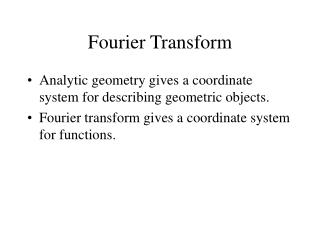DownloadDownload PresentationFourier Transform

# Fourier Transform

Download Presentation## Fourier Transform

- - - - - - - - - - - - - - - - - - - - - - - - - - - E N D - - - - - - - - - - - - - - - - - - - - - - - - - - -
##### Presentation Transcript

1. Fourier Transform • Analytic geometry gives a coordinate system for describing geometric objects. • Fourier transform gives a coordinate system for functions.

2. Decomposition of the image function The image can be decomposed into a weighted sum of sinusoids and cosinuoids of different frequency. Fourier transform gives us the weights

3. Basis • P=(x,y) means P = x(1,0)+y(0,1) • Similarly:

4. Orthonormal Basis • ||(1,0)||=||(0,1)||=1 • (1,0).(0,1)=0 • Similarly we use normal basis elements eg: • While, eg:

5. 2D Example

6. Why are we interested in a decomposition of the signal into harmonic components? Sinusoids and cosinuoids are eigenfunctions of convolution Thus we can understand what the system (e.g filter) does to the different components (frequencies) of the signal (image)

7. Convolution Theorem • F,G are transform of f,g ,T-1 is inverse Fourier transform • That is, F contains coefficients, when we write f as linear combinations of harmonic basis.

8. Fourier transform often described by magnitude ( ) and phase ( ) In the discrete case with values fkl of f(x,y) at points (kw,lh) for k= 1..M-1, l= 0..N-1

9. 11 10 0 0 1 10 X X X X X X F 10 1 0 1 9 11 10 X X 10 1 10 9 0 2 1 1 1 I X X 11 10 9 9 11 1 1 10 1 X X 9 10 11 9 99 11 1 1 1 X X 10 9 9 11 10 10 X X X X X X Remember Convolution O 1/9 1/9.(10x1 + 11x1 + 10x1 + 9x1 + 10x1 + 11x1 + 10x1 + 9x1 + 10x1) = 1/9.( 90) = 10

10. Examples • Transform of box filter is sinc. • Transform of Gaussian is Gaussian. (Trucco and Verri)

11. Implications • Smoothing means removing high frequencies. This is one definition of scale. • Sinc function explains artifacts. • Need smoothing before subsampling to avoid aliasing.

12. Example: Smoothing by Averaging

13. Smoothing with a Gaussian

14. Sampling

15. Sampling and the Nyquist rate • Aliasing can arise when you sample a continuous signal or image • Demo applet http://www.cs.brown.edu/exploratories/freeSoftware/repository/edu/brown/cs/exploratories/applets/nyquist/nyquist_limit_java_plugin.html • occurs when your sampling rate is not high enough to capture the amount of detail in your image • formally, the image contains structure at different scales • called “frequencies” in the Fourier domain • the sampling rate must be high enough to capture the highest frequency in the image • To avoid aliasing: • sampling rate > 2 * max frequency in the image • i.e., need more than two samples per period • This minimum sampling rate is called the Nyquist rate